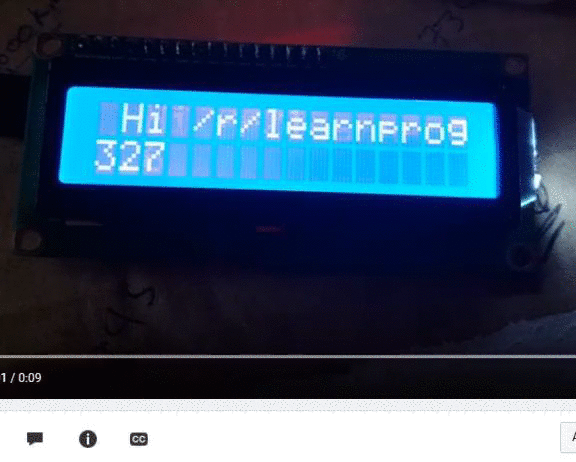# Scrolling text effect on 2D array

For a project I am working on I would like to be abbe to “scroll” an array like so:Here is the code I have so far:

```private boolean[][] display = this.parseTo8BitMatrix("Here");
private int scroll = 0;

public void scroll() {
this.scroll++;
}

//sets the clock's display
public void setDisplay(String s) {
this.display = this.parseTo8BitMatrix(s);
}

//determines the current frame of the clock
private boolean[][] currentFrame() {
boolean[][] currentFrame = new boolean;
int length = this.display.length;
if(length == 32) { //do nothing
currentFrame =  this.display;
} else if(length <= 24) { //center
for(int i = 0; i < 8; i++) {
for(int j = 0; j < length; j++) {
currentFrame[i][j+((32-length)/2)] = this.display[i][j];
}
}
this.display = currentFrame; //set display to currentFrame so the display doesn't get centered each time
} else { //scroll
for(int i = 0; i < 8; i++) {
for(int j = 0; j < length; j++) {
if(this.scroll+j <= 32) {
currentFrame[i][j] = this.display[i][j+this.scroll];
} else {
//?
}
}
}
}
return currentFrame;
}
```

The code I have is effective up until the the array needs to “wrap around” to the other side. Where have I gone wrong?

```currentFrame[i][j]= this.display[i][(j+this.scroll)%length];